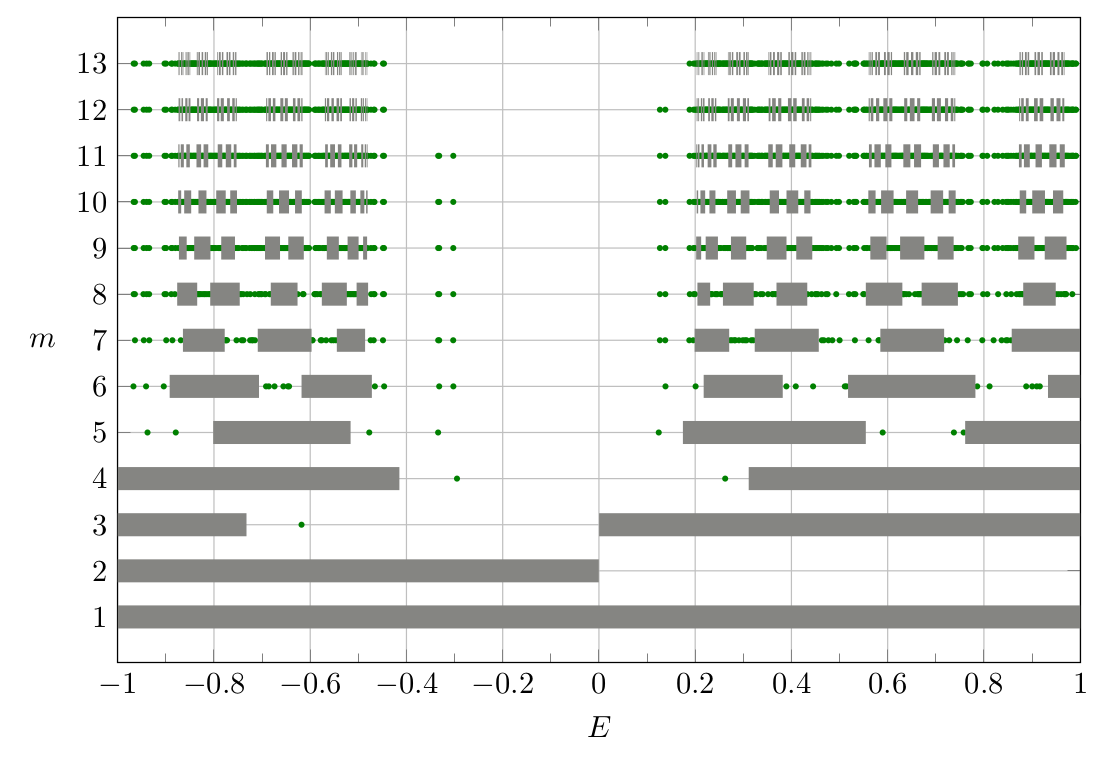TUHH / Institut für Mathematik / Forschungsgebiete / Finite Sections of Aperiodic SchrĂ¶dinger Operators# Finite Sections of Aperiodic SchrĂ¶dinger Operators

## Description

Discrete SchrĂ¶dinger operators are used to describe physical systems on lattices and, therefore, play an important role in theoretical solid-state physics. For a fixed $$p \in [1,\infty]$$, consider the SchrĂ¶dinger operator $$H \colon \ell^p(\mathbb{Z}) \to \ell^p(\mathbb{Z})$$ given by

$(H x)_n = x_{n + 1} + x_{n - 1} + v(n) x_nn \in \mathbb{Z},$(1)

and its one-sided counterpart $$H_+ \colon \ell^p(\mathbb{N}) \to \ell^p(\mathbb{N})$$ given by

$(H_+ x)_n = x_{n + 1} + x_{n - 1} + v(n) x_n\;,n \in \mathbb{N}, \quad x_0 = 0\;.$ (2)

Based on Definitions $$(1)$$ and $$(2)$$, one can associate $$H$$ and $$H_+$$ with infinite tridiagonal matrices $$A = (a_{ij})_{i,j \in \mathbb{Z}}$$ and $$A_+ = (a_{i,j})_{i,j \in \mathbb{N}}$$.

Looking at the corresponding infinite linear system of equations

$A x = b \quad\text{and}\quad A_+ y = c$

it is interesting to know if the solutions $$x$$ and $$y$$ to theses systems can be computed approximately by solving the large but finite linear systems

$A_m x^{(m)} = b^{(m)} \quad\text{and}\quad (A_+)_m y^{(m)} = c^{(m)}$

and letting $$m \to \infty$$. This is the main idea of the Finite Section Method (FSM). In order to assure the applicability of the above procedure, one investigates further properties of the operator $$A$$, the sequence $$(A_n)$$ and its one-sided counterparts. In particular, Fredholm Theory, spectral theory and the concept of limit operators play a central role in this investigation .

This research project deals with the investigation of the applicability of the FSM to problems surging from discrete SchrĂ¶dinger Operators and its interplay with spectral theory, see our works [1-5]. A famous example for theses operators is the so called Fibonacci-Hamiltonian, see , where the potential $$v$$ is given as

$v(n) := \chi_{[1 - \alpha, 1)}(n \alpha \operatorname{mod} 1)\;, \quad n \in \mathbb{Z}.$

For this particular example, the central objects of investigation are periodic approximations $$(A_m)$$. It is crucial to assure that the spectrum of these approximations eventually avoids the point $$0$$ for larger numbers of $$m$$. The following graph shows approximations of the spectra of the one-sided Fibonacci Hamiltonian on $$\ell^2(\mathbb{N})$$.F. Gabel, D. Gallaun, J. GroĂźmann, M. Lindner, and R. Ukena. Analysis Code for Finite Sections of Periodic SchrĂ¶dinger Operators. Publisher: TUHH UniversitĂ¤tsbibliothek. 2021. doi:10.15480/336.3828.

 F. Gabel, D. Gallaun, J. GroĂźmann, M. Lindner, and R. Ukena. Finite Sections of Periodic SchrĂ¶dinger Operators. To appear in: Operator Theory and Applications. 2021. arXiv:2110.09339v2.

 F. Gabel, D. Gallaun, J. GroĂźmann, and R. Ukena. Finite section method for aperiodic SchrĂ¶dinger operators. 2021. arXiv:2104.00711v1.

 F. Gabel, D. Gallaun, J. GroĂźmann, M. Lindner, and R. Ukena. Spectral Analysis Code for Aperiodic SchrĂ¶dinger Operators. Zenodo. 2021. doi:10.5281/zenodo.5201653.

 F. Gabel, D. Gallaun, J. GroĂźmann, and R. Ukena. Spectral approximation of generalized SchrĂ¶dinger operators via approximation of subwords. 2022. 2209.11613v1.

 M. Lindner. Infinite Matrices and their Finite Sections. Frontiers in Mathematics. BirkhĂ¤user Basel, 2006. doi:10.1007/978-3-7643-7767-0.